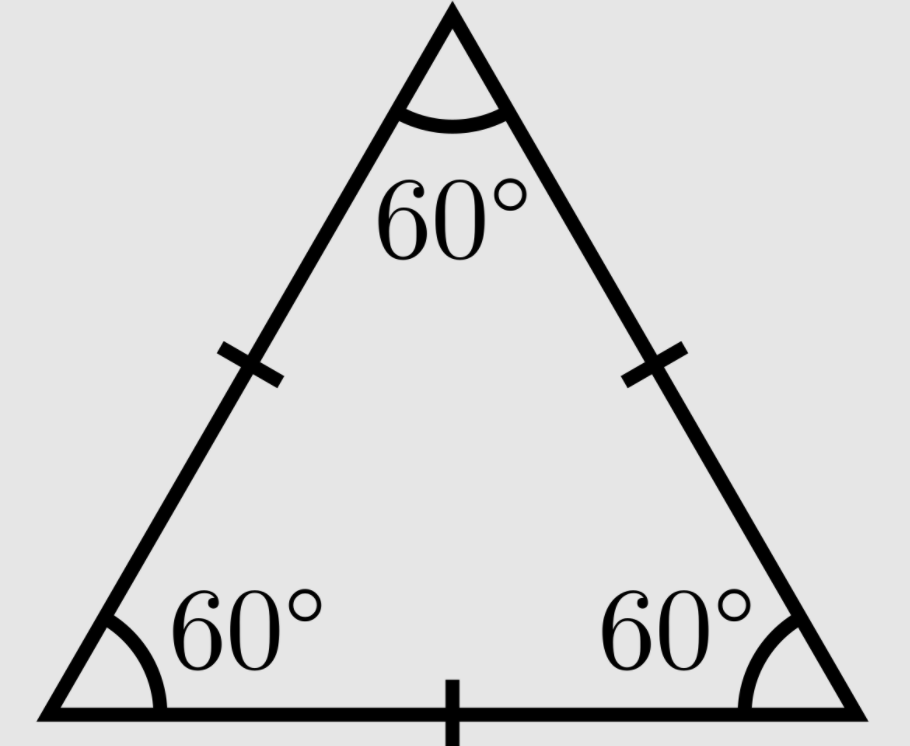100

32 x 2

64

100

How many sides does a quadrilateral have?

4

100

1/4 + 2/4=

3/4

100

96 divided by 6

16

100

What type of angle is this angle?Obtuse

100

Which is larger an yard or a centimeter?

Yard

200

324 x 6=

1,944

200

What kind of triangle is this?Acute Triangle

200

2/3 = ?/12

8

200

24 divide 8

3

200

2,400 divided by 2

1,200
200

What is the best tool to use to measure the width of your desk - a ruler or a scale?

Ruler

300

23 x 33

759

300

What type of angle is 90 degrees?

Right

300

Which of the following fractions is EQUIVALENT to 2/3?

3/4

6/8

5/6

6/9

6/9

300

34 divide 6 =

5 R 4

300

What do you call an answer to a multiplication equation.

The Product.

300

Taylor's grandmother needs 6 liters of milk for a recipe.  Taylor buys her grandmother 600 milliliters of milk.  Did she buy enough milk?

*1 liter = 1,000 mL

No

400

10,457 x 9 =

94, 113

400

A square has one side that is 43 cm long.  What is the PERIMETER of the square?

172 cm

400

1/2 + 2/6=

5/6

400

124 divide 4 =

31

400

4,027-2,738=?

1,289

400

Which is more 3 feet or 42 inches?

42 inches

600

112,789 x 8 =

902,312

600

A square has one side that is 16 cm long.  What is the AREA of the square?

256 square cm

600

convert 18/5 to a mixed number

3 3/5

600

6,437 divided by5

1,287 R2

600

134 x 24

3,216

600

Jessenia's backpack weighs 5 lbs. Karla's backpack weighs 9 lbs.  How many more OUNCES is Karla's backpack than Jessenia's?

1 lb = 16 oz

Karla's backpack weighs 64 oz more.

Click to zoom